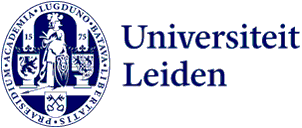Research programme

Statistical Science

The research programme Statistical Science is concerned with the analysis and interpretation of masses of data, the quantification of uncertainty using probability models, and the development and benchmarking of algorithms and methods with these aims.

Contact
Johannes Schmidt-Hieber

The statistical science group investigates methods of data analysis across a full spectrum, from fundamental to applied and multidisciplinary, from model-based to data-driven, and from mathematical to algorithmic. Data science is a modern term for statistical science, albeit that the statistical science group is driven by modelling and analysis and less by collecting, cleaning or storing of data. Theoretical research concerns Bayesian methods, neural networks, uncertainty quantification, multivariate analysis, classification and clustering, statistical learning and information theory, online optimisation and prediction, network analysis, survival analysis, causality and semiparametric models. Application areas include genomics, metabolics, oncology, survival analysis, causality, social science and finance, among many other data-rich areas, where the group works closely with subject matter scientists. The following are some examples of research interests.

The Bayesian statistical method assigns probabilities to the free parameters describing the phenomenon under investigation, reflecting prior uncertainty, and next updates these using the available data. The way of thinking is as simple as it is attractive, but 15 years ago its validity when applied to modern high-dimensional data or infinite-dimensional models was still completely unclear. The statistical science group is a world leader in clarifying aspects of the Bayesian method and its many variants, and is also engaged in applications of such methods to e.g. genomical data.

Modern statistical methods are often computer-intensive, and statistics is intimately connected to machine learning. The statistical science group tries to validate machine learning methods by mathematical theorems, usually stated in terms of probability and risk bounds. For instance, why does a deep neural network work well, or what is a good strategy for online optimization and prediction, e.g. of energy demand?

When data consists of labels rather than numbers, it is often beneficial to transform them before looking for relationships. The statistical science group designs optimal scaling algorithms within the context of multivariate data analysis. One highlight is the package Categories, authored by the group.

The statistical science group participates in the Leiden Center of Data Science and cooperates with statisticians in other faculties in the Leiden Institute for Statistical Science.